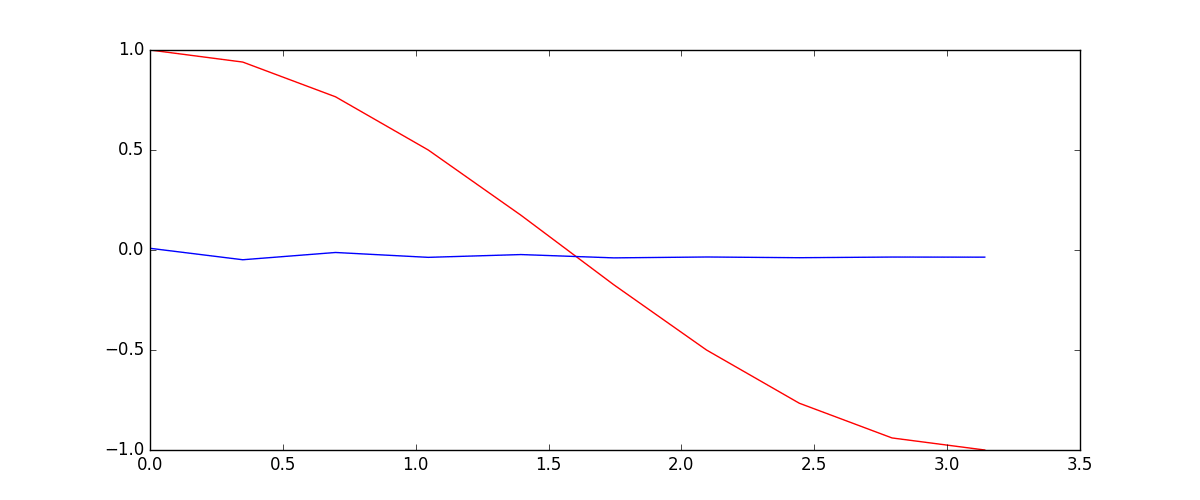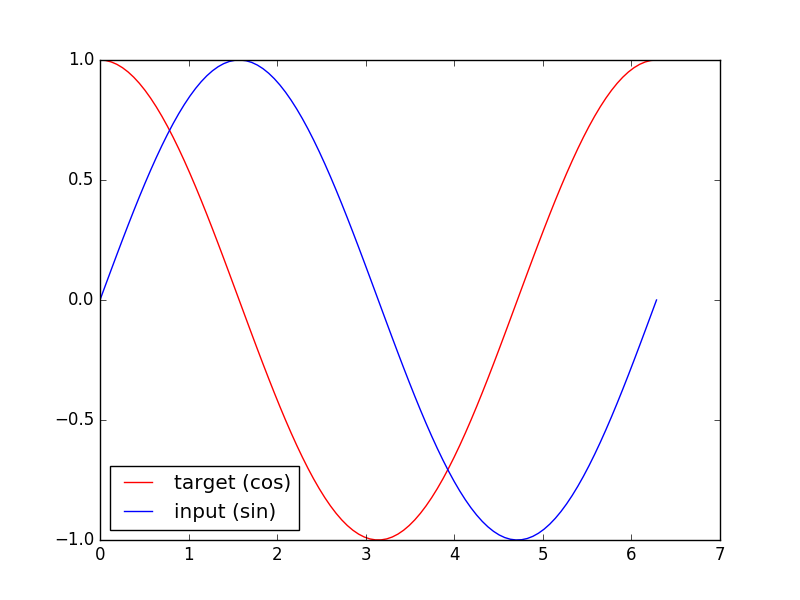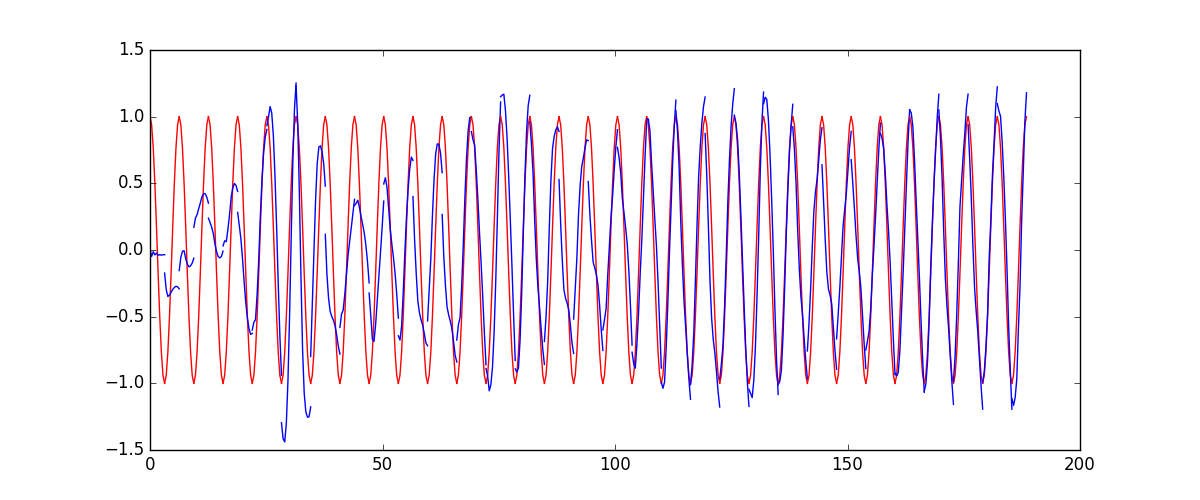#### 学习资料:

RNN 循环神经网络 (回归)：#### 训练数据``````import torch
from torch import nn
import numpy as np
import matplotlib.pyplot as plt

torch.manual_seed(1)    # reproducible

# Hyper Parameters
TIME_STEP = 10      # rnn time step / image height
INPUT_SIZE = 1      # rnn input size / image width
LR = 0.02           # learning rate
``````

#### RNN模型

``````class RNN(nn.Module):
def __init__(self):
super(RNN, self).__init__()

self.rnn = nn.RNN(  # 这回一个普通的 RNN 就能胜任
input_size=1,
hidden_size=32,     # rnn hidden unit
num_layers=1,       # 有几层 RNN layers
batch_first=True,   # input & output 会是以 batch size 为第一维度的特征集 e.g. (batch, time_step, input_size)
)
self.out = nn.Linear(32, 1)

def forward(self, x, h_state):  # 因为 hidden state 是连续的, 所以我们要一直传递这一个 state
# x (batch, time_step, input_size)
# h_state (n_layers, batch, hidden_size)
# r_out (batch, time_step, output_size)
r_out, h_state = self.rnn(x, h_state)   # h_state 也要作为 RNN 的一个输入

outs = []    # 保存所有时间点的预测值
for time_step in range(r_out.size(1)):    # 对每一个时间点计算 output
outs.append(self.out(r_out[:, time_step, :]))

rnn = RNN()
print(rnn)
"""
RNN (
(rnn): RNN(1, 32, batch_first=True)
(out): Linear (32 -> 1)
)
"""
``````

``````def forward(self, x, h_state):
r_out, h_state = self.rnn(x, h_state)
r_out_reshaped = r_out.view(-1, HIDDEN_SIZE) # to 2D data
outs = self.linear_layer(r_out_reshaped)
outs = outs.view(-1, TIME_STEP, INPUT_SIZE)  # to 3D data
``````

#### 训练``````optimizer = torch.optim.Adam(rnn.parameters(), lr=LR)   # optimize all rnn parameters
loss_func = nn.MSELoss()

h_state = None   # 要使用初始 hidden state, 可以设成 None

for step in range(60):
start, end = step * np.pi, (step+1)*np.pi   # time steps
# sin 预测 cos
steps = np.linspace(start, end, 10, dtype=np.float32)
x_np = np.sin(steps)    # float32 for converting torch FloatTensor
y_np = np.cos(steps)

x = Variable(torch.from_numpy(x_np[np.newaxis, :, np.newaxis]))    # shape (batch, time_step, input_size)
y = Variable(torch.from_numpy(y_np[np.newaxis, :, np.newaxis]))

prediction, h_state = rnn(x, h_state)   # rnn 对于每个 step 的 prediction, 还有最后一个 step 的 h_state
# !!  下一步十分重要 !!
h_state = Variable(h_state.data)  # 要把 h_state 重新包装一下才能放入下一个 iteration, 不然会报错

loss = loss_func(prediction, y)     # cross entropy loss